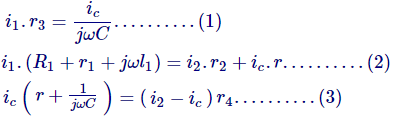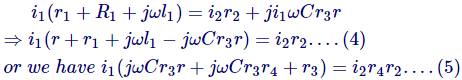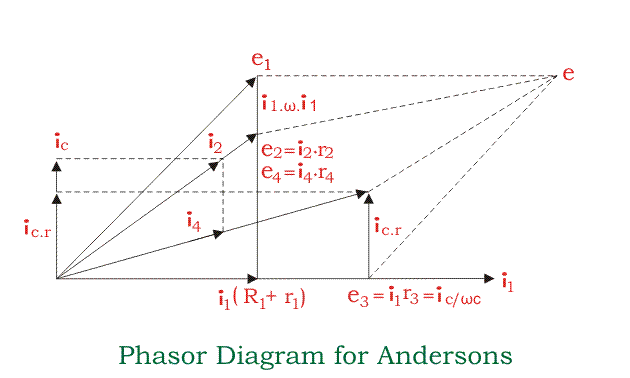## Anderson’s Bridge

Let us understand why there is a need for Anderson’s bridge though we have Maxwell bridge and Hay’s bridge to measure the quality factor of the circuit. The main disadvantage of using Hay’s bridge and Maxwell bridge is that they are unsuitable for measuring the low-quality factor.

However, Hay’s bridge and Maxwell bridge are suitable for measuring accurately high and medium quality factors respectively. So, there is need of bridge which can measure low-quality factor and this bridge is modified Maxwell’s bridge and known as Anderson’s bridge.

Actually, this bridge is the modified Maxwell inductor capacitance bridge. In this bridge double balance can be obtained by fixing the value of capacitance and changing the value of electrical resistance only.

It is well known for its accuracy of measuring inductors from a few micro Henry to several Henry. The unknown value of the self inductor is measured by the method of comparison of the known value of electrical resistance and capacitance. Let us consider the actual circuit diagram of Anderson’s bridge (see figure given below).In this circuit, the unknown inductor is connected between the point a and b with electrical resistance r1 (which is pure resistive).

The arms bc, cd and da consist of resistances r3, r4 and r2 respectively which are purely resistive. A standard capacitor is connected in series with variable electrical resistance r and this combination is connected in parallel with cd.

A supply is connected between b and e.
Now let us derive the expression for l1 and r1:

At the balance point, we have the following relations that hold good and they are:Now equating voltages drops we get,Putting the value of ic in the above equations, we getThe above equation (7) obtained is more complex than we have obtained in the Maxwell bridge. On observing the above equations we can easily say that to obtain convergence of balance more easily, one should make alternate adjustments of r1 and r in Anderson’s bridge.

Now let us look at how we can obtain the value of unknown inductors experimentally. At first set the signal generator frequency at audible range. Now adjust r1 and r such that the phones give a minimum sound.

Measure the values of r1 and r (obtained after these adjustments) with the help of a multimeter. Use the formula that we have derived above in order to find out the value of unknown inductance. The experiment can be repeated with the different values of the standard capacitor.

## Phasor Diagram of Anderson’s Bridge

Let us mark the voltage drops across ab, bc, cd, and ad as e1, e2, e3 and e4 as shown in the figure above.Here in the phasor diagram of Anderson’s bridge, we have taken i1 as the reference axis. Now ic is perpendicular to i1 as the capacitive load is connected at ec, i4 and i2 are lead by some angle as shown in the figure.

Now the sum of all the resultant voltage drops i.e. e1, e2, e3, and e4 is equal to e, which is shown in phasor diagram. As shown in the phasor diagram of Anderson’s bridge the resultant of voltages drop i1 (R1 + r1) and i1.ω.l1 (which is shown perpendicular to i1) is e1. e2 is given by i2.r2 which makes angle ‘A’ with the reference axis.

Similarly, e4 can be obtained by voltage drop i4.r4 which is making angle ‘B’ with the reference axis.

1. It is very easy to obtain the balance point in Anderson’s bridge as compared to Maxwell bridge in the case of low-quality factor coils.
2. There is no need for a variable standard capacitor is required instead of thin a fixed value capacitor is used.
3. This bridge also gives accurate results for the determination of capacitance in terms of inductance.

1. The equations obtained for the inductor in this bridge are more complex as compared to Maxwell’s bridge.
2. The addition of capacitor junction increases complexity as well as the difficulty of shielding the bridge.

Considering above all the advantages and disadvantages, the Maxwell bridge is preferred over Anderson’s bridge whenever the use of a variable capacitor is permissible.

Want To Learn Faster? 🎓
Get electrical articles delivered to your inbox every week.
No credit card required—it’s 100% free.